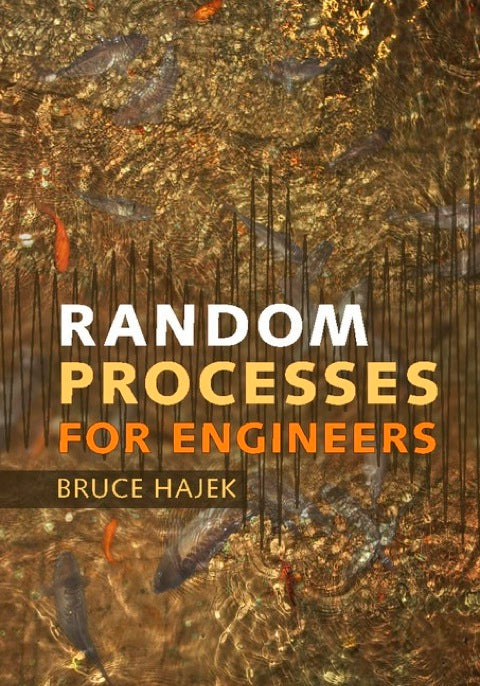# Random Processes for Engineers

Bruce Hajek   ·  ISBN 9781316235577Zookal account needed
Get it instantly
\$80.30  Save \$3.99
\$76.31
 Publisher Cambridge University Press Author(s) Bruce Hajek Published 03122015 Related course codes
This engaging introduction to random processes provides students with the critical tools needed to design and evaluate engineering systems that must operate reliably in uncertain environments. A brief review of probability theory and real analysis of deterministic functions sets the stage for understanding random processes, whilst the underlying measure theoretic notions are explained in an intuitive, straightforward style. Students will learn to manage the complexity of randomness through the use of simple classes of random processes, statistical means and correlations, asymptotic analysis, sampling, and effective algorithms. Key topics covered include: • Calculus of random processes in linear systems • Kalman and Wiener filtering • Hidden Markov models for statistical inference • The estimation maximization (EM) algorithm • An introduction to martingales and concentration inequalities. Understanding of the key concepts is reinforced through over 100 worked examples and 300 thoroughly tested homework problems (half of which are solved in detail at the end of the book).